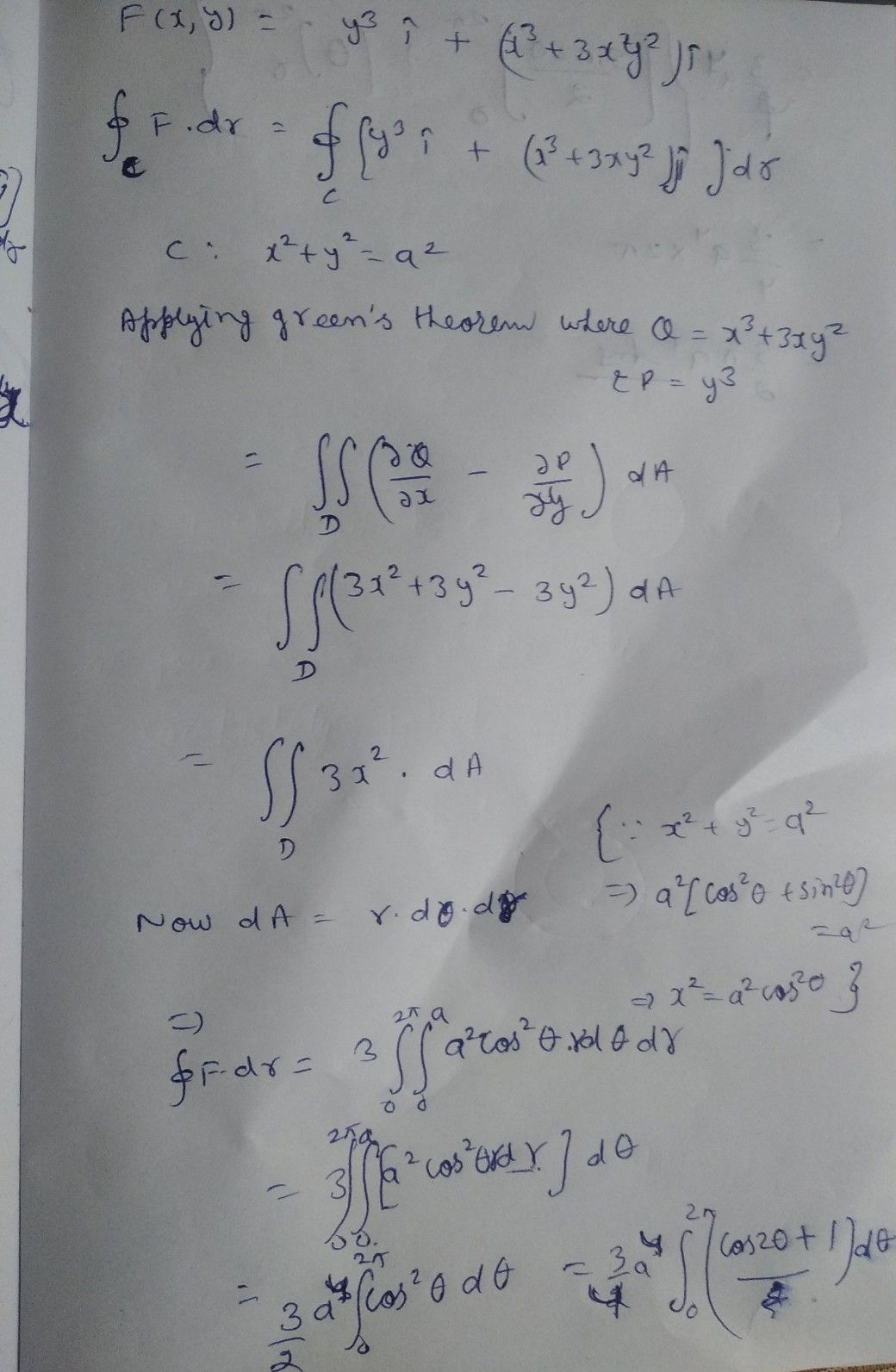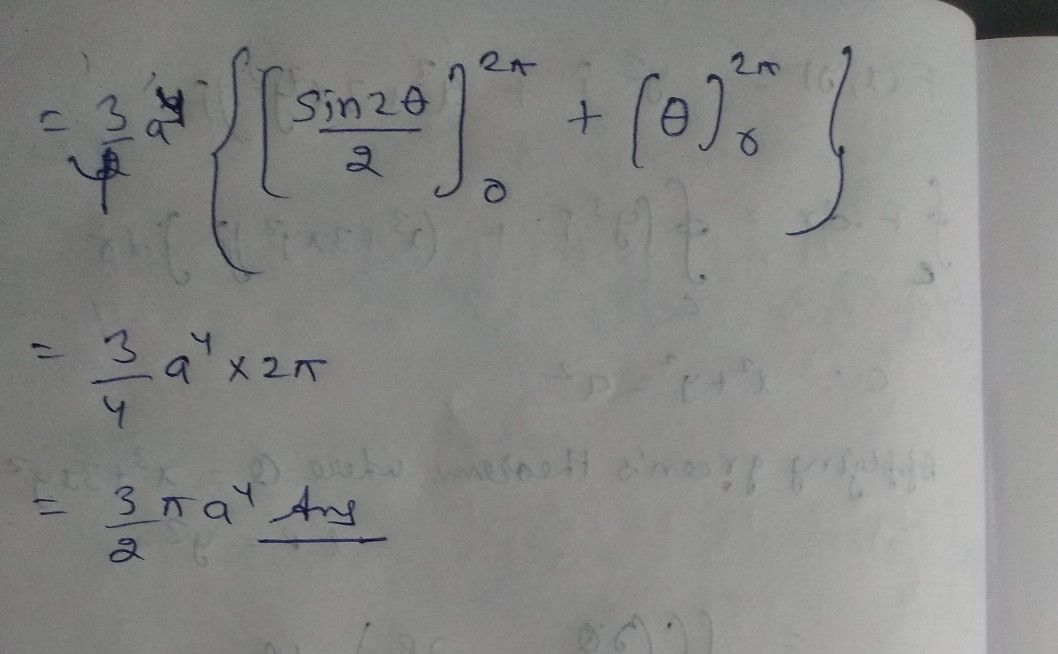Symbol
ProblemUse Green's Theorem to find the work done by the force field $F\left(x,y\right)=y^{3}i+\left(x^{3}+3xy^{2}\right)jln$ moving a particle once in a $C$ counter clockwise direction around the circle of radius $a$ "a" $2$ centred at the origin.
Other
Search count: 106
SolutionQanda teacher - LovishStudent
thanks sir